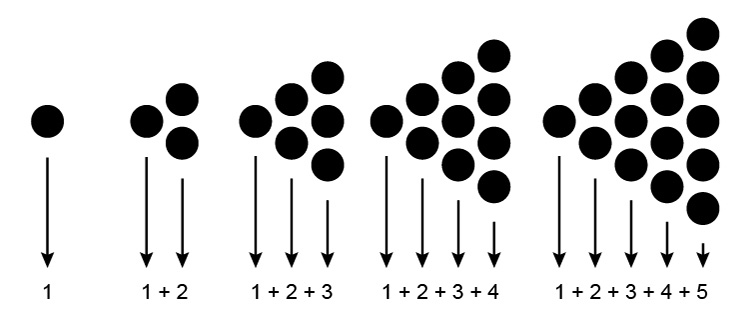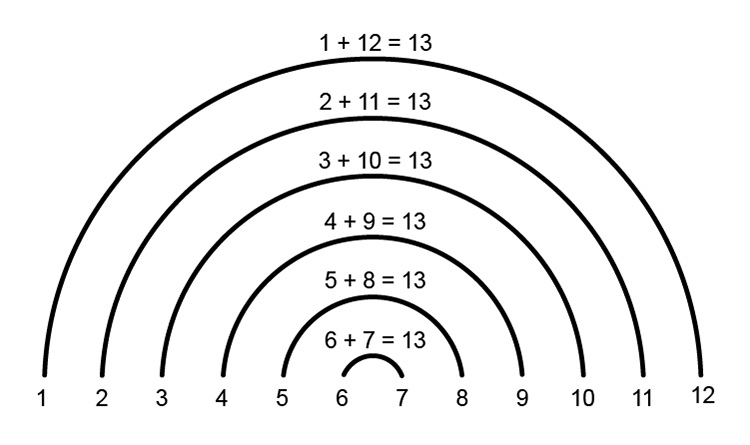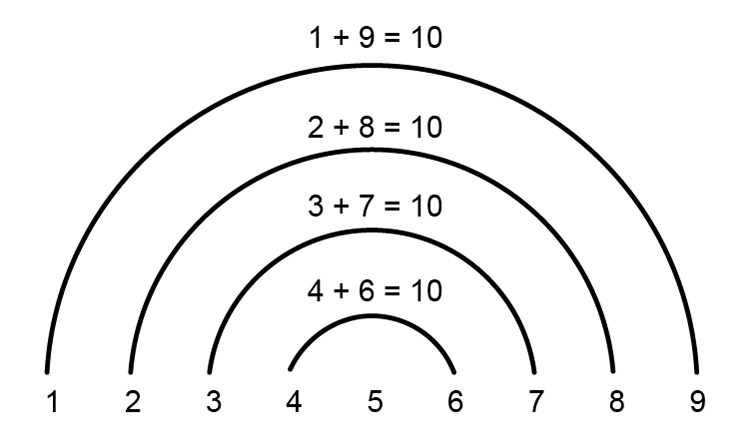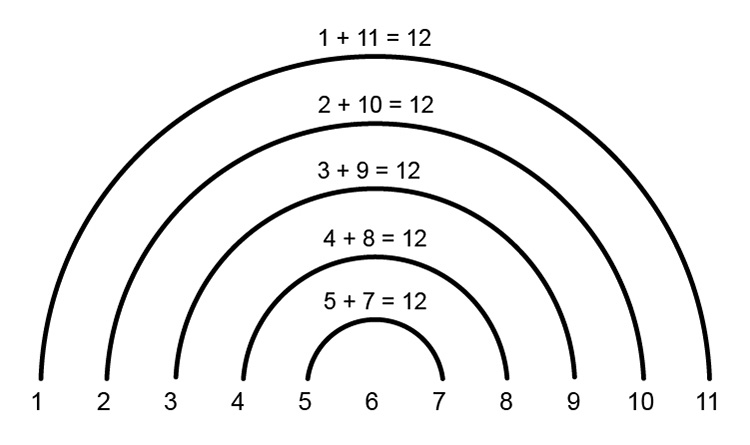# Triangles and the n^(th) term

1   3   6   10   15

You can recognise a triangular sequence by picturing it as follows:We can see a pattern emerge

 1^(st) term =1 =1 2^(nd) term =1+2 =3 3^(rd) term =1+2+3 =6 4^(th) term =1+2+3+4 =10 5^(th) term =1+2+3+4+5 =15

This also gives us a clue as to how we find the n^(th)  term of any triangular sequence (But note the following only applies when the sequence starts at the number 1).

## At first, just take one large even set of n^(th)  terms

Try 12^(th) term

The 12^(th) term would be

1+2+3+4+5+6+7+8+9+10+11+12=78

But a mathematician found that if you started by adding the 1^(st) and last term together and then continue this arrangement then a pattern emerges.

i.e.12^(th) term= 1+12 =13 2+11 =13 3+10 =13 4+9 =13 5+3 =13 6+7 =13 6times13 =78

So the pattern is 13 and the multiple is 6.

This works for any even numbered triangular number.

Try 16^(th) term

 16^(th)  term= 1+16 =17 2+15 =17 3+14 =17 4+13 =17 5+12 =17 6+11 =17 7+10 =17 8+9 =17 8times17 =136

So the pattern is 17 and the multiple is 8.

Now we can form a formula for this

### Stage 1 find the pattern number

12^(th) term =(12+1)=13

16^(th) term =(16+1)=17

So the pattern number =(n^(th)\ \n\u\m\b\e\r+1)

### Stage 2

And the amount of times we repeat this pattern number (the multiple)

12^(th) term =(12)/2=6

16^(th) term =(16)/2=8

So the multiple =(n^(th)\ term)/2

Put stage 1 and stage 2 together we get:

(n^(th)\ term+1)times(n^(th)\ term)/2

So the n^(th)\ term=(n+1)timesn/2

And amazingly this also works for odd triangular numbers too.

Example 1

What is the 9^(th) term of the triangular sequence

Either draw it9^(th) term= 1+9 =10 2+8 =10 3+7 =10 4+6 =10 4times10 +5=45

NOTE:

We add 5 because that is the number missed in the series of semi-circles above.

or using the formula

(n+1)timesn/2

 9^(th)  term =(9+1)times9/2 =(10times9)/2 =5times9 =45

Example 2

What is the 11^(th) term of the triangular sequence

Either draw it11^(th) term= 1+11 =12 2+10 =12 3+9 =12 4+8 =12 5+7 =12 5times12 +6=66

NOTE:

We add 6 because that is the number missed in the series of semi-circles above.

or using the formula

(n+1)timesn/2

 11^(th)  term =(11+1)times11/2 =(12times11)/2 =6times11 =66

Example 3

What is the 27^(th) term of the triangular sequence

We are not going to draw this out but we can just rely on the formula.

(n+1)timesn/2

 27^(th)  term =(27+1)times27/2 =(28times27)/2 =14times27 =378

Example 4

What is the 28^(th) term of the triangular sequence

Again we are not going to draw this out but the formula gives:

(n+1)timesn/2

 28^(th)  term =(28+1)times28/2 =(29times28)/2 =29times14 =406

(As a point of interest comparing example 3 and the answer 378 and here 406 the difference is 28 which would be correct.)